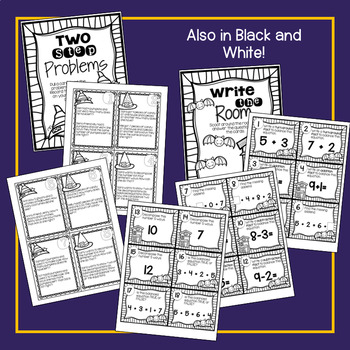# Two Step Word Problems Math Center! With Bonus Review Scoot!Subject
Resource Type
File Type

PDF

(5 MB|21 pages)
Product Rating
Standards
• Product Description
• StandardsNEW

The ability to complete multistep problems is a very useful skill throughout all your life. Exposing students to these types of word problems develops higher order thinking skills and prepares them for more rigorous math later on. This math center helps with practicing this skill in a fun, seasonal way!

BONUS: There's also a balancing facts and basic facts Review Scoot Game included!

To prepare center 1: Make copies of recording page per student. Laminate and cut apart cards.. Place in folder with recording pages at math centers.

To prepare center 2: Make copies of recording page per student. Laminate and cut apart cards. Hang cards around the room. Place recording pages in folder and store in centers location.

*PLEASE NOTE: These centers can be found bundled in my Balancing Facts Math Centers! product.

Included:

• Two Step Problems (T)
• Write the Room (M)
• Center Game cover pages
• Accountability sheets

All center materials in black and white, too!

This math center is designed to complement my MATH! Curriculum for first grade. You can find it HERE.

These are my Math Tubs:

M – Model It!

A – Add or Subtract It!

T – Think It!

H – Hone It!

IT – I’m Through!

You can read about how I set up my Math Centers HERE.

Thanks and happy teaching!!

Jessica

Like Tech and Teachability on Facebook

Fluently add and subtract within 20 using mental strategies. By end of Grade 2, know from memory all sums of two one-digit numbers.
Understand the meaning of the equal sign, and determine if equations involving addition and subtraction are true or false. For example, which of the following equations are true and which are false? 6 = 6, 7 = 8 - 1, 5 + 2 = 2 + 5, 4 + 1 = 5 + 2.
Add and subtract within 20, demonstrating fluency for addition and subtraction within 10. Use strategies such as counting on; making ten (e.g., 8 + 6 = 8 + 2 + 4 = 10 + 4 = 14); decomposing a number leading to a ten (e.g., 13 - 4 = 13 - 3 - 1 = 10 - 1 = 9); using the relationship between addition and subtraction (e.g., knowing that 8 + 4 = 12, one knows 12 - 8 = 4); and creating equivalent but easier or known sums (e.g., adding 6 + 7 by creating the known equivalent 6 + 6 + 1 = 12 + 1 = 13).
Relate counting to addition and subtraction (e.g., by counting on 2 to add 2).
Apply properties of operations as strategies to add and subtract. If 8 + 3 = 11 is known, then 3 + 8 = 11 is also known. (Commutative property of addition.) To add 2 + 6 + 4, the second two numbers can be added to make a ten, so 2 + 6 + 4 = 2 + 10 = 12. (Associative property of addition.)
Total Pages
21 pages
N/A
Teaching Duration
N/A
Report this Resource to TpT
Reported resources will be reviewed by our team. Report this resource to let us know if this resource violates TpT’s content guidelines.